# Geospatial Big Data Processing with Python: Detecting Green Roofs in Toronto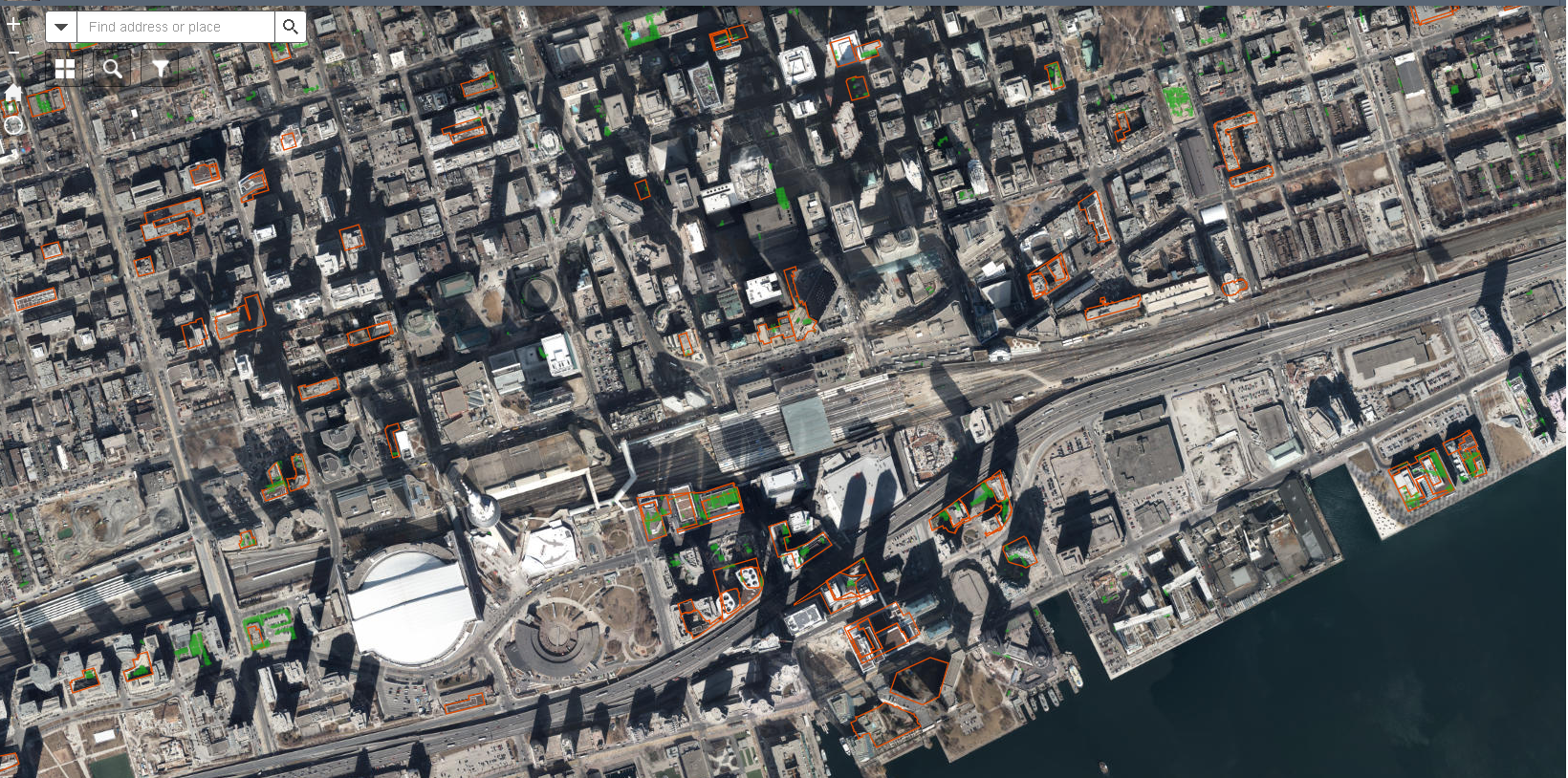Detecting Green Roofs in Toronto

# Introduction

Detecting green roofs is a complicated process due to the impact of tree-shade on building rooftops in satellite images. The vegetation index is an indicator that describes the greenness — the relative density and health of vegetation — for each picture element, or pixel, in a satellite image. One of the most widely used indicators is the Normalized Difference Vegetation Index (NDVI). NDVI values range from +1.0 to -1.0. In most cases, NDVI values between 0.2 and 0.4 correspond to areas with sparse vegetation; moderate vegetation tends to vary between 0.4 +and 0.6; anything above 0.6 indicates the highest possible density of green leaves.

# Datasets

1. Building Data: 3D Massing 2019 Shapefile (City of Toronto’s Open Data Portal)

2. Digital Globe Satellite Images (2018–07–10T16:27:14 private)

# Image Processing: 1.3 Billion Points

Let’s plot the raster data and check its size.

`plt.figure(figsize=(12, 8))data.plot()# plotting takes time due to large file size`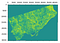Raster

The cell size of the raster is 1 m x 1 m and the raster data has 42335 columns by 30696 rows. Each cell size represents 1m². The total number of records (cells in the raster file) is 1.3 billion(1293491700). GeoRasters can easily convert a raster file to a DataFrame easily as below:

`df = data.to_pandas()`

However, the raster data used in the above-mentioned example is too large to be converted to a DataFrame using GeoRasters. Reading such large data into pandas can be difficult, especially if we work on a personal computer. So it is necessary to reduce the size of the data. Let’s convert the raster data to CSV points and remove NoData values from the raster to reduce its size.

`# Converting raster to csv. this process takes time.from osgeo import gdalfrom osgeo import gdalconstInRaster = r”downloads\ndvi_merged\merged.tif”OutCSV = r"D:\downloads\ndvi_merged\test2.csv"# open the raster and get propertiesds = gdal.OpenShared(InRaster,gdalconst.GA_ReadOnly)GeoTrans = ds.GetGeoTransform()ColRange = range(ds.RasterXSize)RowRange = range(ds.RasterYSize)rBand = ds.GetRasterBand(1) # first bandnData = rBand.GetNoDataValue()if nData == None: nData = -9999else: print(“NoData value is {0}”.format(nData))HalfX = GeoTrans / 2HalfY = GeoTrans / 2with open(OutCSV,’w’) as CSVwrite: for ThisRow in RowRange: RowData = rBand.ReadAsArray(0,ThisRow,ds.RasterXSize,1) for ThisCol in ColRange: if RowData[ThisCol] != nData: if RowData[ThisCol] > 0: X = GeoTrans + ( ThisCol * GeoTrans ) Y = GeoTrans + ( ThisRow * GeoTrans )  X += HalfX Y += HalfY CSVwrite.write(‘{0},{1},{2}\n’.format(X,Y,RowData[ThisCol]))`

Let’s check the size of the CSV data.

`size= os.path.getsize(r”D:\downloads\ndvi_merged\test2.csv”)print(‘File size: ‘ + str(round(size / (1024 * 1024 * 1024), 3)) + ‘ Gigabytes’)`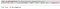CSV File Size

Let’s check desktop memory.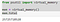Desktop memory

# Dask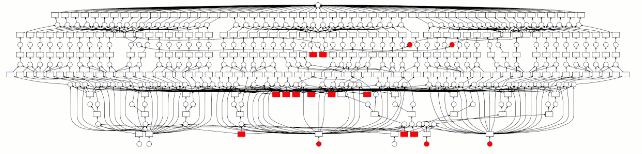Dask: Scale up to clusters

Dask provides advanced parallelism for analytics, enabling performance at scale for the popular python tools Numpy, Pandas, and Scikit-Learn.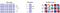https://dask.org/

⮚ Dask arrays scale Numpy workflows, enabling multi-dimensional data analysis in earth science, satellite imagery, genomics, biomedical applications, and machine learning algorithms.

⮚ Dask DataFrames scale Pandas workflows, enabling applications in time series, business intelligence, and general data munging on big data.

⮚ Dask-ML scales machine learning APIs like Scikit-Learn and XGBoost to enable scalable training and prediction on large models and large datasets.

Let’s check Machine Specifications.

Dask’s read_csv takes no time at all.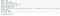Reading CSV

With 33.4GB of data, we can see it takes a few seconds to get the result. Let’s check the DataFrame.

`df_tmp`
`import timestart = time.time()import dask.dataframe as ddimport multiprocessingimport dask_geopandas as dg# dask’s read_csv takes no time at all!ddf = dd.read_csv(r”D:\downloads\ndvi_merged\test2.csv”, names=[‘lng’, ‘lat’, ‘ndvi’])end = time.time()print(end — start)#0.3410015106201172`

Let’s check the DataFrame size. It has more than 624.68 million records

Let’s convert data types of Dask DataFrame to speed up data processing.

`ddf[[‘lng’,’lat’,’ndvi’]]=ddf[[‘lng’,’lat’,’ndvi’]].astype(‘float32’)`

There are 560 partitions in the DataFrame. Let’s check the first partition of the DataFrame:

`# get first partitionpart_0= ddf.get_partition(0)part_0.tail()`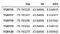The first partition of dataframe

Let’s filter green NDVI points with NDVI values greater than 0.4 to reduce the DataFrame size and to get green NDVI points.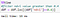Filtering DataFrame

Let’s check DataFrame size:

The filtered NDVI data is as below: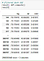Filtered DataFrame

The filtered NDVI data has around 300 million points.

# Building Data

`# download building dataimport zipfileimport iofrom pyproj import Proj, CRS, transformurl = ‘https://ckan0.cf.opendata.inter.prod-toronto.ca/download_resource/09197dd4-f440-49c0-acc5-41e2585ef9f7'local_path = ‘./tmp’print(‘Downloading zipped shapefile…’)r = requests.get(url)z = zipfile.ZipFile(io.BytesIO(r.content))print(“Done”)z.extractall(path=local_path) # extract to tmp folderfilenames = [y for y in sorted(z.namelist()) for ending in [‘dbf’, ‘prj’, ‘shp’, ‘shx’] if y.endswith(ending)] print(filenames)shp= gpd.read_file(r’./tmp/3DMassing_2019_WGS84.shp’)shp.head(5)`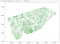Toronto Building Data

# Spatial Join

It took 2 minutes to merge 300 million NDVI points with the building data. Let’s check spatially joined DataFrame.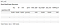Spatially joined Dask DataFrame

The DataFrame has 560 partitions. Let’s check the first partition of the DataFrame.

`part_0= ddf_out.get_partition(0)part_0.tail()`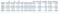Spatially joined NDVI data

Let’s create NDVI Geopandas DataFrame.

`points = ddf_out.compute()crs = CRS(“EPSG:3857”)points_gdf = gpd.GeoDataFrame(points, crs = crs, geometry=’geom’)points_gdf.head(10)`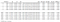NDVI point data

It can be seen from the above DataFrame that there are 7.33 million NDVI points joined with building data. Let’s check the result and group NDVI points by building to measure rooftop green area size. Each NDVI point represents 1 m² green roof area.

`#groupby building_id and count ndvi values by  using dask%timeresult = ddf_out.groupby(ddf_out.building_id).ndvi.count().compute()`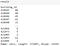Grouped NDVI Data by Buildings

Let's merge the result with building data to get building geometry.

`merged = gpd.GeoDataFrame(pd.merge(result.to_frame(), shp, left_index=True, right_on='building_id'))merged`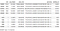Building NDVI data

It can be seen from the above result that, the merged data has 421096 building polygons with NDVI values. The NDVI count value represents the size of rooftop green area (m²) in the result. However, it is necessary to filter buildings with high NDVI count value and roof area to eliminate the tree shadow effect. Let’s plot the result data to check the building polygons with NDVI data.

`ax = points_gdf.plot(figsize=(15, 15), markersize =1)shp.plot(ax=ax,edgecolor=’cyan’, facecolor = ‘white’, linewidth =1)points_gdf.plot(ax = ax,color=’green’, markersize =1)ax.set(xlim=(-8849000, -8842000), ylim=(5421000, 5423500))`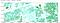Building Polygons with NDVI data

# Zonal Statistics and Spatial Join in ArcPro

`%%timeimport arcpyimport pandas as pdfrom arcgis.features import GeoAccessor, GeoSeriesAccessorfrom arcgis.features import SpatialDataFramefrom arcpy import envimport numpy as npimport os,sysarcpy.ia.ZonalStatisticsAsTable(“building4326”, “FID”, “ndvi_merged_4326.tif”, r”C:\Users\gis\Documents\ArcGIS\Projects\deep\deep.gdb\ZonalStat”, “DATA”, “ALL”, “CURRENT_SLICE”, 90, “AUTO_DETECT”)ZonalStat= arcpy.management.AddJoin(“building4326”, “FID”, “ZonalStat”, “FID”, “KEEP_ALL”)def table_to_data_frame(in_table, input_fields=None, where_clause=None):    """Function will convert an arcgis table into a pandas dataframe with an object ID index, and the selected    input fields using an arcpy.da.SearchCursor."""    OIDFieldName = arcpy.Describe(in_table).OIDFieldName    if input_fields:        final_fields = [OIDFieldName] + input_fields    else:        final_fields = [field.name for field in arcpy.ListFields(in_table)]    data = [row for row in arcpy.da.SearchCursor(in_table, final_fields, where_clause=where_clause)]    fc_dataframe = pd.DataFrame(data, columns=final_fields)    fc_dataframe = fc_dataframe.set_index(OIDFieldName, drop=True)    return fc_dataframezonalstat = table_to_data_frame("building4326")zonalstat.head(5)#Wall time: 4min 28s`

# GeoPandas and RasterStats

`%%timefrom rasterstats import zonal_statsbldg1 =gpd.read_file(r"C:\Users\gis\Documents\tmp\building4326.shp")bldg1['count'] = pd.DataFrame(    zonal_stats(        vectors=bldg1['geometry'],         raster=r"D:\downloads\ndvi_merged\ndvi_merged_4326.tif",         stats='count'    ))['count']`

Processing large amounts of data (1.3 billion points) in ArcPro is also very difficult. We preferred to use the previous Dask data processing approach to create a more accurate green rooftop layer and to measure green rooftop size.

We filtered the top 1000 buildings with high NDVI values, large rooftop area, and the tallest buildings with high NDVI values.

`filtered = merged.nlargest(1000,[‘ndvi’,’avg_height’,’poly_area’])filtered.head(20)`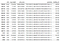Filtered Buildings

Let’s plot the first building to see if it is accurate.

`shp_165816 = shp.loc[(shp[‘building_id’]==165816)] points_165816 = points_gdf.loc[(points_gdf[‘building_id’]==165816)]ax = points_165816.plot(figsize=(15, 15), markersize =1)shp_165816.plot(ax=ax,edgecolor=’red’, facecolor = ‘white’, linewidth =1)points_165816.plot(ax = ax,color=’green’, markersize =1)`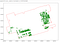The First Building with Green Roof

It is visible that we got accurate green roof data. The first building (‘building_id’]==165816) has 12142 m² green rooftop area. Let's plot the filtered top 1000 buildings with folium to check the result.

`def embed_map(m): from IPython.display import IFrame m.save(‘index.html’) return IFrame(‘index.html’, width=’100%’, height=’750px’)m = folium.Map(location=[43.6532, -79.3832], zoom_start=12, tiles=’http://services.arcgisonline.com/arcgis/rest/services/World_Imagery' + ‘/MapServer/tile/{z}/{y}/{x}’, attr=’Ablajan or anything else…’)building = filtered.to_crs(epsg=’4326').to_json()style = {‘fillColor’: ‘#006400’, ‘lineColor’: ‘#228B22’}building = folium.features.GeoJson(building, style_function=lambda x:style)m.add_child(building)results =”C:\\Users\\gis\\Desktop\\”m.save(os.path.join(results, ‘building.html’))embed_map(m)`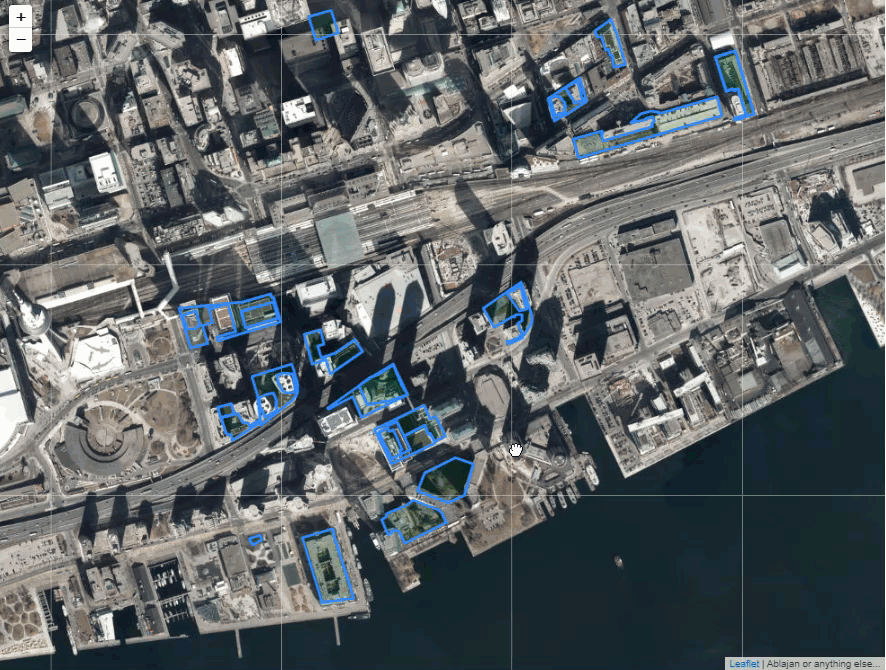Plotting Green Roofs with Folium

# Machine Learning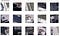Single-Shot Detector (SSD)

The sample test of the Mask R-CNN model is as below:

`# Load a random image from the images folderIMAGE_DIR=r”C:\dev\timg”file_names = next(os.walk(IMAGE_DIR))image = cv2.imread(os.path.join(IMAGE_DIR, random.choice(file_names)))# # load model weightsmodel_path = r’C:\Users\gis\Downloads\greenroof\gr_cfg20191111T1809\mask_rcnn_gr_cfg_0005.h5'class_names = [‘BG’, ‘greenroof’]model.load_weights(model_path, by_name=True)# Run detectionresults = model.detect([image], verbose=1)# Visualize resultsr = resultsvisualize.display_instances(image, r[‘rois’], r[‘masks’], r[‘class_ids’], class_names, r[‘scores’])`

We found that this approach requires more training and testing data to achieve a high accuracy rate ( Mask R-CNN and Deep Learning (fast.ai )notebooks are not posted here).

In the end, we detected and verified more than 600 buildings with green roofs. The final result is displayed on the following map (The map can be accessed at this link https://webmap.ca/sn/greenroofs.html)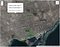Toronto Green Roofs

# Conclusion

I’d like to give a big thanks to my colleagues: Karl Merrem, Zivorad Djokic, George Epure, and Bruce Walker for geocoding Green Roof permit data, image processing, and for verifying green roof results. My appreciation also goes to my former colleague Matthew Tenney for initiating the Green Roof project and to Ryan Garnett for providing 2018 Digital Globe Satellite Images for this Project.

Please don’t hesitate to write comments and questions.

## References:

Senior Geospatial Specialist in Toronto

## More from Ablajan Sulaiman

Senior Geospatial Specialist in Toronto